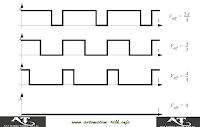## Using Analog Output in PLC Programming

In the Previous article we discussed about Analog Inputs In PLC and tried to understand the basic concepts and converting of analog Value in PLC. Similarly today we will learn about the Analog Outputs in PLC , how to use it and the how internally analog value is calculated in PLC.

### Analog Output Processing in PLC :-

Generally we use analog output of PLC to operate all devices which work on Analog Input such as 0 to 10 v signal for VFD or 0 to 10 V signal for any control Valve. As with the analog Inputs we can similarly get either Voltage or current Output from PLC depending on the requirement.
To set an analog Output from PLC an Integer is converted to a respective Voltage.The process of this conversion is very fast and does not have any significant time delay but the Problem of quantization errors exists here. In the Internal circuit of the PLC , a D/A converter is there for converting the Integer to Analog Value.

### Quantization Error in Analog Output of PLC:-

Let us try to understand that how the quantization error generates. Suppose we have a PLC which has 8 bit D/A converter which gives 0 to 10 V Output. The resolution of 8 bit D/A converter will be 256 , so 0 will result in 0 Volt and 255 will result in 10V. Suppose we want an output of 6.234V so we will specify the Integer Value of 159. But if we calculate inversely than this integer value will result in 6.235V , so the quantization error would be 6.235V-6.234V=0.001V.

### Generating Analog Output Using PWM Outputs :-

Below you can see the PWM Waveforms.Pulse Width Modulation Outputs can also be modified to generate the Analog Output , but these will output only a fixed Voltage or 0 Volt. To obtain a value in between the Max and Min of voltage , the voltage is quickly switched on and Off to reduce the effective voltage. The switching of Duty Cycle determines the Effective Voltage Output.

For the latest updates you can subscribe.Subscribe to Automation-Talk by Email.